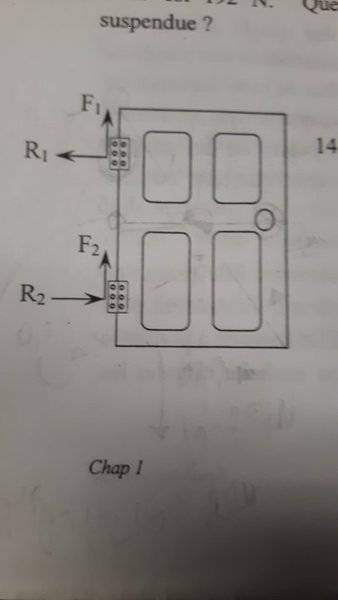# What is the force exerted by each hinge on the door?1. Homework Statement

The door has a mass of 30 kg, is 200 cm long and 90 cm wide. The hinges are installed at 25 cm from the bottom (R2) and 25 cm from the top (R1). What are is the magnitude of the force exerted by each hinge on the door? The door is in a state of static equilibrium.

## Homework Equations

Torque = rFsinθ
∑Torque = 0 Nm
∑F = 0
Fg = 30kg*9.8m/(s^2) = 294 N

## The Attempt at a Solution

a) Place pivot midway between hinges.
⇒F1 and F2 exert no torque (Parallel to axis or rotation).
⇒ F1+F2 = Fg = 294 N. No way to find each force individually.

⇒Lever arm for R1 = 0.75 m, θ = 90°; same for R2
Since ∑Torque = 0 Nm; Torque by R1 = Torque by R2 (opposite sign), so they must exert the same force (opposite sign) on the door.

STUCK. A hint please? Thanks!

## Answers and Replies

I thought it might have been easier to place the "pivot" on one of the hinges.

TSny
Homework Helper
Gold Member
Since ∑Torque = 0 Nm; Torque by R1 = Torque by R2 (opposite sign), so they must exert the same force (opposite sign) on the door.
It appears that you are assuming that the only forces that exert torques about your chosen pivot point are the forces R1 and R2. Would the weight force exert a torque about this pivot point?

I agree with Tom that placing the pivot point at one of the hinges is a good choice.

Keep in mind that ΣF = 0 applies to both the horizontal components and to the vertical components of the forces. That is, you have two equations ΣFx = 0 and ΣFy = 0.

So I just tried placing the pivot at the upper hinge and I think I got it:

Torque of Fg = 0.45m*294N*sin90°=132.3 Nm
Torque of Fg is opposed by Torque of R2, so Torque R2 = 132.3 Nm, lever arm = 1.5m, so R2 = 88.2 N = R1

Thanks a lot :)